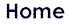Logic and Philosophy of Logic Super-Induction Method: Logical Acupuncture of Mathematical Infinity A. Zenkin Computing Center of the Russian Academy of Sciences, Moscow, Russia alexzen@com2.com.ruABSTRACT: The logical legitimacy problem of computer proofs related to the well-known Horgan-Kranz discussion (published in Scientific American (1993), Notices of AMS (1996), etc.) is considered in this paper. The new logical-mathematical method — Super-Induction Method — for proving common mathematical statements by means of a computer is described. The main features of this method are: 1) an analytical mathematical proof of an unusual reliable inference 'from a single to a common' of the form "IF there exists n* such that Q(n*) holds THEN for all n>n* P(n) is true", where Q and P are some number-theoretical predicates, and 2) a reduction of the proof of the common mathematical statement "P(n) for all n greater than or equal to n*" to a computer searching of a unique single natural number n* (a unique acupuncture point of the infinite natural number series) which possesses a unique collection of number-theoretical properties Q(n*). If such the acupuncture number n* is found, then we can prove the common statement "P(n) for all n greater than or equal to 1", possibly, except for some n less than or equal to n*. Using a so-called Cognitive Computer Graphics (CCG) Visualization of abstract number-theoretical objects, the proof can be reduced in many cases to a demonstration of the corresponding CCG-pictures: the strict mathematical proof is reduced to a visually ostensive one. One of such ostensive proofs of real number-theoretical theorems is given. Relations of the super-induction method to other known ones are briefly discussed.I. Introduction Any non-trivial mathematical discovery always has non-trivial philosophical and psychlogical aspects. Mathematicians during a lot of centures discovered irrational numbers, infinitesimals, uncoutable sets, etc. Modern philosophy discusses today epistemological problems of commensurability/incommensurability, finitness/infinitness, discreteness/continuity, etc. Mathematics is a unique mean for the world cognition. The main problem of cognitive psychology is the process itself of the human-being cognition. So, the process of mathematical discovery is one of the most important object for cognitive psychology investigations. In the paper, a new technology of mathematical discoveries is discribed. These discoveries were obtained by means of a direct visual contact of a human-being with visual semantical images of abstract mathematical objects. All these mathematical discoveries themselves, and especially the process iteslf of their obtaining are very non-trivial, and represent a fruitful ground for philosophical and psychological investigations. II. A New Kind of Mathematical Reasonings In 1949, German mathematician H. E.Richert proved  one unusual statement as to mathemantical properties of the common finite natural numbers. Unfortunately, he (and nobody after him) did not understand the epistemological sense of his own theorem. In 1978-1979, I discovered two new different classes of the alike statements called later as EA-Theorems thanks to their special logical structure [2,3]. These statements are a new type of mathematical and logical reasoning. Consider the common series of natural numbers,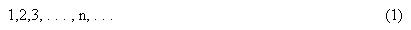and let P(n) and Q(n) are two number-theoretical properties (predicates) given on (1). Then, the H.E.Richert's and two our Theorems have the following quite unusual form. EA-THEOREM. IF there EXISTS AT LEAST ONE natural number, say n*, such that a predicate Q(n*) holds, THEN FOR ALL natural numbers n > n* a predicate P(n) is true, or, in the short symbolic form: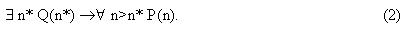So, EA-Theorem states an absolutely "impossible" thing: the COMMON statement "n>n*P(n) is rigorously DEDUCED from a SINGLE statement \$n*Q(n*). In other words, the Theorem is an AUTHENTIC DEDUCTION of the "from a SINGLE to a COMMON" INDUCTION. Such the statements might seem to be a nonsense from the modern meta-mathematics and mathematical logic point of view (a corresponding discussion see in ), but these EA-Theorem are mathematical Theorems and they are proved by the Classical Mathematics methods, i.e., absolutely rigorously. In particular, the logical and mathematical correctness of the H. E. Richert's EA-Theorem is certifying not only by Richert's proof itself, but also by the following historical fact having the important cognitive significance. Original H. E.Richert's paper was published in 1949 , and then it was described almost word for word in the page 143 of famous W.Sierpinski's monography "Elementary Theory of Numbers" . From its appearance moment (1964), the W.Sierpinski's book became a handbook and a manual for 99% of mathematicians, and a lot of thousands, or even millions, of mathematicians had been reading the page 143 with H. E.Richert's Theorem from 1964 up to now. But none of them payed attention to that unusual EA-Theorem just in the connection with the epistemology of the inductive inference. Using his EA-Theorem, H.E.Richert proved a lot of beautiful new theorems in classical Number Theory on representations of natural numbers by sums of different prime numbers. So, the EA-Theorems are really a totally new kind of inductive statements in Mathematics and Logic. Some important and unexpected consequences, beyond the bounds of only pure mathematics, follow from such the EA-Theorems. II. "Generalization" of J. S. Mill's Inductive Logic As is known, the most fundamental paradigm of the Classical Mill's Inductive Logic sounds so: It is always possible to formulate (not to deduce, but, rather, to invent ) a common statement (and even not one) from a set of particular facts. But such the statement will always be a plausible, hypotetical statement only, and never an authentic one, i.e., any induction of the kind "from a particular statment to a common one" is always a plausible induction, and never a realiable one. The Paradigm is the corner-stone of all the Natural Science. So, the EA-Theorems prove mathematically, i.e., rigorously in the calssical logic sense, the authentic induction (2) "from a SINGLE fact to a COMMON statement." Of course, EA-Theorems refer (today) to some areas of Number Theory and discrete mathematics only, and therefore it does not disprove the Mill's paradigm in general. But it brings to light some new and unexpected (including, first of all, philosophical) aspects of our true cognition of the Inductive Logic paradigm. III. Generalization of the Most Famous Deductive Rule of Aristotle's Classical Logic The question is about the famous modus ponens rule of Aristotle's Logic. As is well known, the rule is formulated as follows: IF a statement A is true and IF the statement A ® B is proved, THEN the statement B is true too. From the Aristotle's time, they have in mind that the statement A ® B is a deductive inference of a less common statement B from a more common statement A, for example, a deductive inference of a theorem from axioms in modern meta-mathematics. The EA-Theorems prove mathematically, i.e., by a rigorously deductive way, that famous Aristotel's modus ponens rule is valid when a statement A is a single statement, but its consequence B is a common one. So, the EA-Theorems generalize the modus ponens rule of Aristotle's Logic to such the "impossible" case. It needs to remark, that the Classical Logic, and even the modern meta-mathematics logic do not forbid such the case explicitly. Simply, they were considering such a case never. IV. Generalization of the Complete Mathematical Induction B. Pascal's Method It is most fantastical that in general case there are no restrictions to number-theoretical predicates P and Q. Indeed, you can take any P and any dependence Q=f(P). The most terrible what can occur is that you simply will not prove the corresponding EA-Theorem, and nothing more. For example, let P be an arbitrary number-theoretical predicate. Then, using our choice freedom, we can define a new predicate Q=f(P), say, as follows: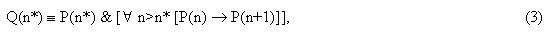where n*-is now a variable natural number. Since n in (3) is a bound variable, our predicate Q(n*) depends really from n* only. So, even by a pure formal way, substituting this Q(n*) in EA-Theorem (2), we obtain: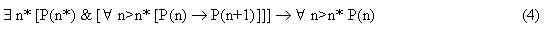As it is easy to see, the expression (4) is the famous Complete Mathematical Induction Method by B.Pascal in its more correct notation than its traditional notation: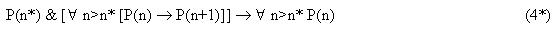REMARK. In tasks that was solved, for example, by H.E.Richert, the threshold number n* may take different values such as 9, 17, 29, and so on. As is well known, as to the Complete Mathematical Induction, the corresponding "theshold" number n* is, usually, equal to either 0 or 1, and very seldom n* is some more than 1 (see corresponding discussion on this theme in ). Today, we already know three absolutely different classes of such the EA-Theorems, and the today's maximal threshold number n*=77 900 162 [2,6]. I think the classical mathematical induction with an alike value for n* is not known (this problem was also discussed at ). The Super-Induction method works fine in such areas of discrete mathematics where the usual mathematical induction method simply does not work. Today, the choice of the predicate Q for a given predicate P, and the formulation of the mathematical connection Q=f(P) in the EA-Theorems do not have a theory, i.e, they are quite "irrational", i.e. pure intuitive, informal, actions which realize a natural human-being's aspiration for an unrestricted freedom for the mathematical creativity, in complete accordance with the famous slogan by George Cantor. Of course, till this aspiration leads us out reasonable frames. So, the EA-Theorems generalize the Classical Complete Mathematical Induction Method which kids of all over the world study at school from the XVII Century. For the very end of XX Century, it is not trivial event having an obvious sense for the Philosophy and Psychology of scientific cognition. Super-Induction Method The Super-Induction (SI) method itself is based on the EA-Theorems and has the following absolutely evident and natural formulation [2,3].is constructed (is devised!), where the number-theoretical predicates Q and P are distinct, i.e. Q = f(P) ¹ P. 3. The EA-statemenet (2) is proved analytically (i.e., as usually, less general statements are deduced from more general ones). 4. The truth of the single statement \$ n*Q(n*) of the (already, after point 3) EA-Theorem (2) is proved; i.e., a natural number n*, posessing the number-theoretical property Q, is found. 5. If we succeed in finding such a unique number n*, then the proved truth of the single statement \$n*Q(n*) and the proved truth of the EA-Theorem \$n* Q(n*) ® "n>n* P(n) implay (by modus ponens) the authentic truth of the general statement "n> n* P(n). 6. The truth of the predicate P(n) is checked for all n £ n*: (a) If "n£n* P(n), then we have proved "n³1 P(n); (b) Otherwise, we find (explicitely!) all elements of the finite exclusive set, N* = { 1 £ n £ n* : ØP (n)}, and thus, 7. We prove our general statement in the form: "n³1 P(n), except for n ÎN*. So, the logical and mathematical sense of the Super-Induction method is demonstrated in Fig 1.I believe, that, say, if A.Wiles proved that the Last Fermat's Theorem is true for all powers r³2 EXCEPT FOR some exclusive set, say, N* of values of r AND enumerated ALL such elements of the set N*, no mathematician would be objecting against the statement that A.Wiles solved (and closed) the problem. Because in Mathematics, to solve a common problem of the form "n³1 P(n) denotes not to prove its true or false, but to indicate excpicitely a set of n where P(n) is true and a set of n where P(n) is false. In this sense, it can be said that the mathematical understanding of the common statement notion generalizes the usual understanding of the common statement notion of Classical Logic. Practical Part Some Words on the Cognitive Computer Graphics. Already quite long ago, we worked out a quasi-"multi-media" system of Cognitive Reality based on the Cognitive Computer Graphics (CCG) conception  Such the CCG-Technique allows a scientist to be plunged into the color-musical world of mathematical abstractions of a high level in order to see, to look at, to touch, and to manipulate them by visual, musical, semantical, aesthetic, and even ethic channels. Such the multi-modal CCG-interaction with scientific problems activates the scientific intuition and the right-hemispheric, visual thinking of a human-being, and essentially extends his creative abilities. A lot of real unique mathematical and logical discoveries was made by means of such the CCG-approach: e.g., generalizations of famous Waring's Problem and D. Hilbert's solution of that Problem, discoveries of new mathematical objects in the very beginning of the common series of Natural Numbers, of a new universal additive property of these Numbers, of a new class of virtual geometrical objects which run and jump (in direct visual sense) along the common series of the Natural Numbers, which (these objects) can be seen in the Cognitive Reality CCG-space only, etc.[7,8] In particual, all results, discussed above, were obtained by means of such the Cognitive Computer Graphics Technique . CCG-Discovery of EA-Theorems In 1770, English mathematician E.Waring formulated his famous problem about representing natural numbers by sums of r-th powers (r³2) of non-negative integers: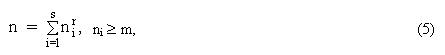where, for Classical Waring's Problem, m = 0, and r ³ 2, s ³ 1-are fixed integers. During more that 200 years, this Classical (m=0) Waring's Problem (CWP) was being investigated by such the outstanding mathematicians as Euler, Lagrange, Gauss, Legandre and others, but it was David Hilbert who first gave the complete solution of CWP in 1909 only (see Table 1, the left column).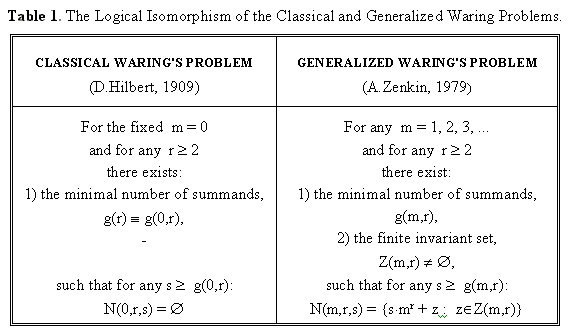Here, for arbitrary values of the parameters m ³ 0, r ³ 2, and s ³ 1, we introduce, by definition, the following two families of sets of natural numbers: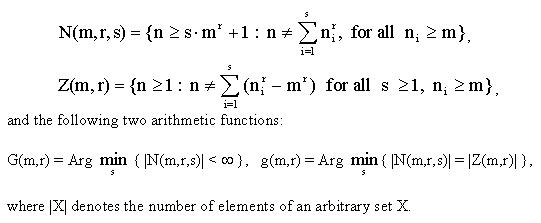The mathematics itself is not the aim of this paper, therefore I shall not specify these terms (see [2, 8-10]), which further will be used only in historical and heuristical aspects. In 1933, American mathematician G.Pall  made the first step towards a non-trivial extension of Classical Waring's Problem, viz he solved that Problem for the simplest non-classical case m=1, r=2. But only half a century later, the next step was made. And that step was accomplished by means of a computer and the CCG-approach. In Fig 2 and 3, that G.Pall's achievement is shown. Remark, that all color-musical images (so-called phythograms) of number-theoretical properties are read (more precisely, are counted) from left-to right and from top to bottom as any usual text page.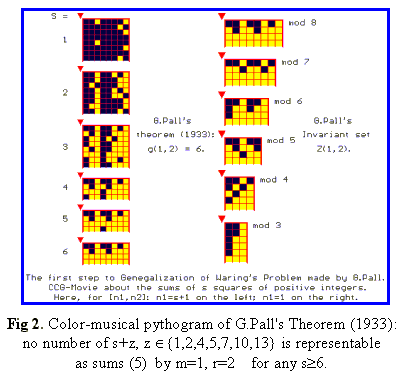In the very beginning of 80s, the CCG-approach allowed us, first, to see a conceptual generalization of CWP (m=0) in the form of a color-musical movie, and then to formulate a so-called Generalized Waring's Problem (GWP) that holds for any m=1,2,3, ... . Some later, the complete solution of GWP was given (see Table 1, on the right) [2,12,13]. In other words, it was just the CCG-approach that allowed to see 1st, 2nd, 3rd and so on (till the infinity) storeys of famous Waring's problem that was considered hitherto as a problem of the 0-floor solely. In order to understand the true significance of the CCG-approach in that generalization, it has to emphasize that Euler, Lagrange, Gauss, Hilbert and others were not only the scientists of genius, but they were the scientists with a genius intuition. But even they could not lift over the 0-storey of the Classical Waring's Problem. It is rather interesting fact just from the point of view of the psychology of scientific cognition and of the role of visual intuitive thinking in scientific discoveries. As is known, all new-fangled Number-Theoretical objects in the XX Century (Cantor's transfinite sets, Suslin's sets, Borel's sets and the like) are placed out of all "VISIBLE" bounds of common finite Natural Numbers: nobody and never had seen such objects. The CCG-visualization allowed us to discover a whole twice infinite family of new number-theoretical objects-these invariant sets, Z(m,r), m=1,2,3,..., r=2,3,4,..., of the Generalized Waring's Problem-which "live" in the very beginning of the usual Series of the common finite Natural Numbers. Some of these new mathematical objects are presented in Fig 3. All these sets consist of a finite quantity of the common natural numbers not exceeding the number 100. It must be emphasized here once more that the question is about the "very finite" objects that are till now unknown in modern Mathematics. It is a good place to emphasize here that, in the classical case m=0, the corresponding invariant sets Z(0,r) are empty for all powers r ³ 2. Possibly, the last is one of the main psychological reasons why such the natural generalization of the Classical Waring's Problem has not attracted an attention of a lot of outstanding Number Theorists during the last two centures. I believe this pure mathematical CCG-facts have also deep sense from the point of view of philosophy and psychology of our scientific cognition. Unfortunately, some leading experts in modern analitical Number Theory believe that only a paper filled in a lot of analytical theory formulas is a science. All other is not a science. Probably, such experts know the Number Theory History quite bad, since such, for example, Number Theory Creators as Pythagoras, Fermate, Euler, Lagrange, Gauss, Weierstrass, Cauchy, Dedekind, etc. did not know the analitical Number Theory. Simply because they lived long before modern mathematicians began to apply complex variable analytical methods in Number Theory. But just they created the Science called Number Theory. As well known, partial differential equations expert Prof. S.G. Krantz  states in his polemics with John Horgan [ 15] that computer calculations can't be arguments in mathematical proofs, and the computer pictures "prove nothing". I agree with Prof. S.G.Krantz, but in general only. Indeed, nobody can guarantee that, for example, the very finite pythograms of the invariant sets Z(m,2), m=1,2,3,4, r=2, presented in Fig. 3, contain all their elements, i.e. that they don't have other elements which are not presented in these computer pictures. In other words, the question is here about a mathematically rigorous VISUAL PROOF of the FINITY of all these invariant sets. In [2,11,12], the general theorem on the finiteness of the invariant sets Z(m,r) was proved for any m³1, r³2. However, in practice, it is frequently more convenient to use the constructive criterion of the finiteness for invariant sets, Z(m,r) for any given values m and r. Such the criterion is given by the next EA-theorem [2,3].PROOF. Full proof is given in [2,3], BTW this proof is carried out by means of the reduction ad absurdum method, and does not use the mathematical induction method. It's important logical moment by virtue of the aforesaid connection between the common induction and the super-induction. Thus, EA-Theorem 1 asserts the validity of the already known us EA-statement: IF there exists (at least, one!) a natural number n*, posessing the given set of the number-theoretical properties Q(n*), THEN any n > n* posesses the corresponding number-theoretical property P(n). Now, according to the Super-Induction method, in order to prove the finiteness of invariant sets by means of the EA-Theorem 1, it is sufficient to find at the pythogram of the corresponding invariant set Z(m,2) such the element zÎZ(m,2),-(here) a yellow square,-which is followed by no less than q(m,2) =(m+1)2 -m2 of (here) black squares (naturally, if such a yellow square exists, since Theorem 1 does not guarantee (!) the existence of such threshold natural numbers). Taking into account, that according to the EA-Theorem condition: q(1,2) = 3, q(2,2) = 5, q(3,2) = 7, q(4,2) = 9, the pythograms of the invariant sets exhibited in Fig. 3 directly VISUALLY implay that: n*(1,2) = 13, n*(2,2) = 28, n*(3,2) = 52, n*(4,2) = 79. Thus, once having found the numbers n*(m,2) for m=1,2,3,4, we proved actually the truth of the singular antecedents of implication (2). From this, by modus ponens rule, we immediately infer that "n>n*P(n),-where, by virtue of the EA-Theorem 1, P(n) = " n Ï Z(m,r) ", and, consequently, really prove that the corresponding invariant sets are finite. What occurs for all n£n*, can be directly seen in the corresponding pythograms. More strictly, we can see (and, if desired, can even write explicitly) all elements of the corresponding invariant sets Z(m,2), m = 1, 2, 3, 4. Here is another example of non-trivial usage of the super-induction method. As is known, towards the middle of 80s, the Classical Waring Problem was solved for all powers r except for the single case r=4. And only in 1986, the famous problem for r=4 was at last solved by R. Balasubramanian, J. Deshouillers, and F. Dress . The patriarch of modern analytical Number Theory, Prof. A. Schinzel, in his traditional ten years review estimated that solution as an outstanding achievement of Number Theory in 80s. All analytical number theorists were agereed with such high estimation. After knowing the result, I "invented" and proved the corresponding EA-Theorem of a new kind. So, it remained only to find a threshold, say, k*(1,4) in order to generalize the R. Balasubramanian, J. Deshouillers, and F. Dress's result to non-classical case m=1. But the searching for the threshold natural number n* occured not a simple matter. During about month, using all possible analytical methods (for shorting the step-by-step examination), Vladimir Vojevodsy (today a well-known mathematician) and I were searching for such k*(1,4) by a computer IBM PC286. Ultimately, we found it: k*(1,4)=77900162. The Super-Induction method allowed immediately to generalize the R. Balasubramanian, J. Deshouillers, and F. Dress's result to the non-classical case of sums of positive (m=1) integers: g(1,4)=21 [2,17,18]. The corresponding invariant set Z(1,4) is presented in Fig 4.I must remark here, that only the top pythgram of the set Z(1,4) was of interest for us. First of all because the EA-Teorem 1 allows to prove visually the finity of the set Z(1,4). Indeed, for m=1, r=4 we have q(1,4) = (1+1)4-14 = 15. From the other hand, we SEE (!) that the "last" yellow number in the pythogram is followed much more than 15 black numbers (one can even not to count such the black numbers!). So, that yellow number is our thershold number N*(1,4). Now, spent 5-7 minutes to count all yellow numbers directly on the pythogram, we obtain: the number of Z(1,4) elements is |Z(1,4)| = 1321 and max{Z(1,4)} = n*(1,4) = 2641. Now the Super-Induction method (checking P(n) for all n < 2641) gives the complete solution of the Generalized Waring's Problem for the non-classical case m=1, r=4. Moreover, just the top pythogram of the invariant set Z(1,4) by the optimal modulus 75 allowed us firstly TO SEE and then TO PROVE some other new mathematical statements [2, 8] Now, as to the lower pythogram of the same set Z(1,4,) by a non-optimal modulus 78. It seems (today) to be less interesting from a mathematical point of view. But I believe it is quite interesting as an artistic aesthetical object of visual mathematics and it is quite attractive from a standpoint of the Mathematical Education-XXI. As to the aesthetics of abstract Mathematical objects you can visit our non-usual "Artistic p-Number Gallery" at . CONCLUSIONS. I would like to emphasize, that in modern mathematics, there are no analogues of the Siper-Induction method. In particular, this method cannot be reduced to the method of common mathematical induction, which, as is known, always deals with one predicate. The SI-method differs essentially from methods based on a direct (analytical!) proof of the existence of a threshold element n0 such that \$n0"n>n0 P(n) (see, e.g., the mentioned above solution of the Classical Waring's Problem for biquadrates, which does not contain the implicative part of the form (2) at all), as well as from methods of classical deduction, which infer a less general statement from more general one and, as a rule, do that without a computer. At the same time, construction (at present, invention!) and an analytical proof of EA-Theorems (2) "from a single statement to a common one", as well as a computer search for a unique threshold number n* followed by computations for n
 References 1. Richert, H.E., Uber Zerlegungen in paarweise vershiedene Zahlen, Norsk Mat. Tidssk. 31 (1949), pp. 120-122. 2. Zenkin A.A., Cognitive Computer Graphics-M.: NAUKA, 1991, 192 pp. 3. Zenkin A.A., Superinduction: A New Method For Proving General Mathematical Statements With A Computer.-Doklady Mathematics, Vol.55, No.3, 410-413 (1997). 4. W.Sierpinski, "Elementary Theory of Numbers" -Warszaw, 1964; pp.143-144. 5. FOUDATION OF MATHEMATICS [FOM] discussion e-group. E- archive and information files are available on the web at: http://www.math.psu.edu/simpson/fom/ 6. Zenkin A.A., Waring's problem: g(1,4) = 21 for fourth powers of positive integers.- Computers and Mathematics with Applications, Vol.17, No. 11, pp. 1503-1506 (1989). 7. Al.&Ant. Zenkins, "VIRTUAL GEOMETRY OF NATURAL NUMBERS" at: 8. Al.&Ant. Zenkins, "COGNITIVE COMPUTER VISUALIZATION" at: 9. Zenkin A.A., Generalized Waring's Problem: An Estimation Of G(m,r)-Function Via g(m-1,r)-Function By Means Of The Superinduction Method.-Doklady Mathematics, vol 56, No. 1, 597-600 (1997). 10. A.A.Zenkin, Generalized Waring's Problem: G(m,r)£ G(0,r) +m+1 for any m ³ 1, r ³ 3.-Doklady Mathematics, vol 56, No. 1, pp. 499-501 (1997). Translated from Doklady Alademii Nauk, Vol 355, No. 2, 1997, pp. 151-153. 11. Pall G., On sums of squares.-Amer. Math. Monthly, 1933, vol.40, 10-18. 12. Zenkin A.A., Generalization of A.Wieferich's Theorem on the Case of Natural Summands.-Doklady AN USSR, 1982, n.264, No.2, 282-285. {Translated in "Sov. Math. Dokl.", Vol. 25, 616-620 (1982).} 13. Zenkin A.A., Some extensions of Pall's theorem.-Computers and Mathematics with Applications, Vol. 9, 609-615 (1983). 14. Krantz S.G. The Immortality of Proof.-Notices of the American Mathematical Society, vol. 41, No.1, pp. 10-13 (1994). 15. John Horgan, The Death of proof.-Scientific American, vol. 269, No.4, pp. 92-103 (1993). 16. Balasubramanian R., Deshouillers J., Dress F., Waring's problem for biquadrates,1:Sketch of the Solution.- Comptes Rendus de l'Academie des Siences, Ser. 1, Math., vol. 303 (4), 85-88 (1986). 17. Zenkin A.A., Waring's problem from the standpoint of the cognitive interactive computer graphics.-Mathematical and Computer Modelling, Vol.13, No. 11, pp. 9-36 (1990). 18. A.A.Zenkin, Generalized Waring's Problem: On One New Additive Property of Natural Numbers .-"Matem. Zametki", TOM 58, No.3, 372-378, 1995 {Translated in: "Mathematical Notes", vol. 58, no. 3, pp.933-937 (1995)}. 19. Al.&Ant. Zenkins, ARTISTIC p-NUMBER GALLERY at http://www.com2com.ru/alexzen/gallery/Gallery.html 20. A.A.Zenkin, Superinduction Method: Logical Acupuncture of Mathematical Infinity.-In the Collection Infinity in Mathematics: Philosophical and Historical Aspects, Edr. Prof. A.G.Barabashev.-Moscow: "Janus-K", 1997, pp. 152-168, 173-176.Paideia logo design by Janet L. Olson. All Rights Reserved